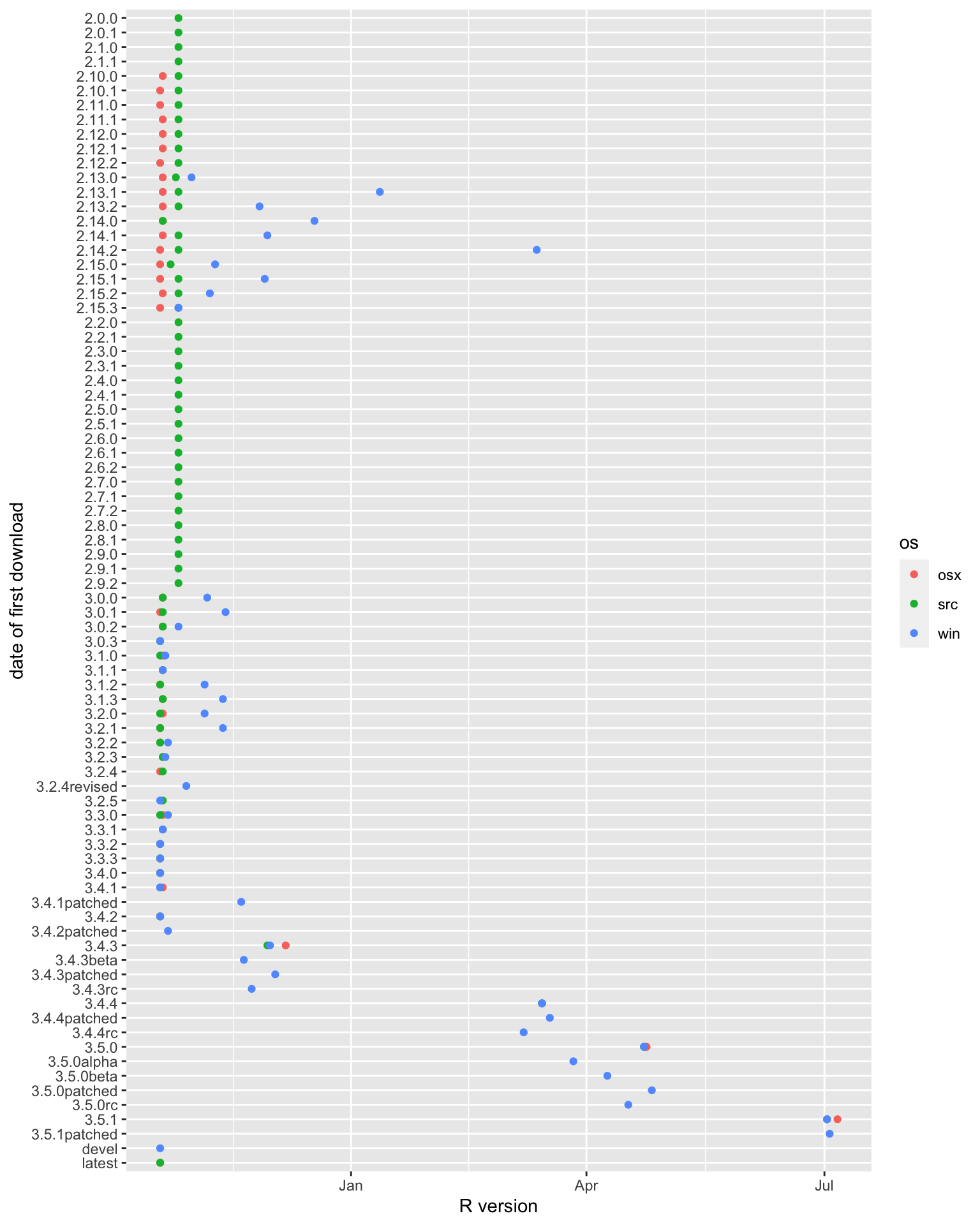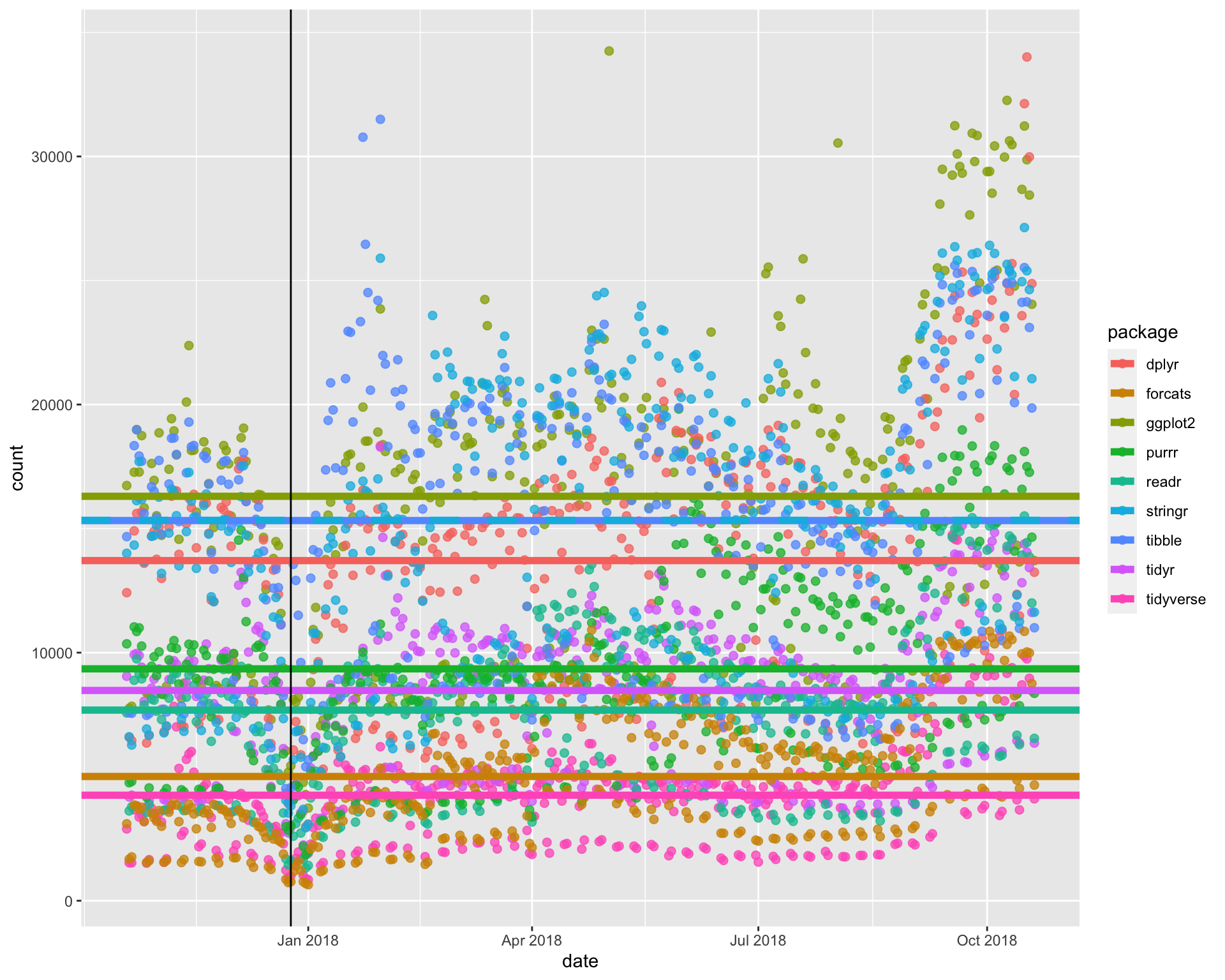Sara Stoudt true
10-30-2018
``````library(ggplot2)
library(dplyr)
library(tidyr)
library(forcats)
library(cranlogs)

setwd("~/Desktop/tidytuesday/data/2018/2018-10-30")
rd\$date <- as.Date(as.character(rd\$date))
``````

``````rd %>%
drop_na(os) %>%
group_by(version, os) %>%
summarise(first = min(date)) %>%
ggplot(., aes(fct_rev(version), as.Date(first), col = os)) +
geom_point() +
coord_flip() +
ylab("R version")
``````Take-Aways

• Windows often lags.
• I suspect “devel” and “latest” are relative to the current version since they appear early on.

### Tidyverse and its components

``````tidyverse <- cran_downloads(package = "tidyverse", from = min(rd\$date), to = max(rd\$date))

allTy <- rbind.data.frame(tidyverse, ggpl, dp, tid, re, pr, tib, st, fc)

## add a horizontal line representing mean, help us pick out different colors
orderV <- allTy %>%
group_by(package) %>%
summarise(mV = mean(count)) %>%
arrange(mV)
``````
``````ggplot(allTy, aes(date, count, col = package)) +
geom_point(size = 2, alpha = .75) +
geom_hline(data = orderV, aes(yintercept = mV, col = package), lwd = 2, lty = c(rep(1, 7), 2, 1)) +
geom_vline(aes(xintercept = as.Date("2017-12-25")))
``````Note: `stringr` and `tibble` are on top of one another.

Take-Aways

• The average number of downloads of `tidyverse` is less than the average number of downloads for each of its components. This makes sense because we can either download all packages at once via `tidyverse` or download the components separately.

• `ggplot2` has the highest average number of downloads.

• `stringr` and `tibble` have similar average number of downloads.

• There is some evidence of a bit of work life balance as there is a noticeable drop off in downloads on Christmas.